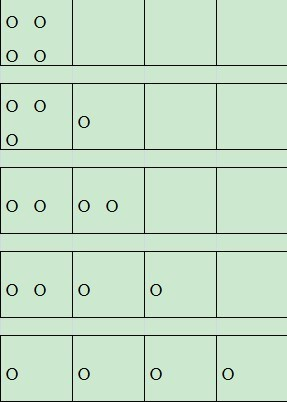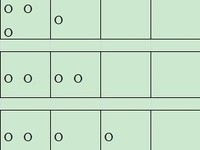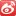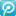• 正整数拆分
2020-10-30 03:15:01

参考博客 :

# 一、正整数拆分基本模型

无序拆分基本模型 :

将 正整数 N N 无序拆分成正整数 , a 1 , a 2 , ⋯   , a n a_1, a_2, \cdots , a_n 是拆分后的 n n 个数 ,

该拆分是无序的 , 上述拆分的 n n 个数的个数可能是不一样的 ,

假设 a 1 a_1 x 1 x_1 , a 2 a_2 x 2 x_2 个 , ⋯ \cdots , a n a_n x n x_n , 那么有如下方程 :

a 1 x 1 + a 2 x 2 + ⋯ + a n x n = N a_1x_1 + a_2x_2 + \cdots + a_nx_n = N

这种形式可以使用 不定方程非负整数解个数 的生成函数计算 , 是 带系数 , 带限制条件的情况 , 参考 : 组合数学】生成函数 ( 使用生成函数求解不定方程解个数 )

无序拆分的情况下 , 拆分后的正整数 , 允许重复 和 不允许重复 , 是两类组合问题 ;

如果不允许重复 , 那么这些 x i x_i 的取值 , 只能 取值 0 , 1 0, 1 ; 相当于 带限制条件 , 带系数不定方程非负整数解 的情况 ;

对应的生成函数是 : G ( x ) = ( 1 + y a 1 ) ( 1 + y a 2 ) ⋯ ( 1 + y a n ) G(x) = (1+ y^{a_1}) (1+ y^{a_2}) \cdots (1+ y^{a_n})

如果 允许重复 , 那么这些 x i x_i 的取值 , 就是 自然数 ; 相当于 带系数不定方程非负整数解 的情况 ;

对应的生成函数是 : G ( x ) = ( 1 + y a 1 + y 2 a 1 ⋯   ) ( 1 + y a 2 + y 2 a 2 ⋯   ) ⋯ ( 1 + y a n + y 2 a n ⋯   ) G(x) = (1+ y^{a_1}+ y^{2a_1}\cdots) (1+ y^{a_2} + y^{2a_2}\cdots) \cdots (1+ y^{a_n}+ y^{2a_n}\cdots )

G ( x ) = 1 ( 1 − y a 1 ) ( 1 − y a 2 ) ⋯ ( 1 − y a n ) G(x) =\cfrac{1}{ (1-y^{a_1}) (1-y^{a_2}) \cdots (1-y^{a_n}) }

# 二、有限制条件的无序拆分

将 正整数 N N 无序拆分成正整数 , a 1 , a 2 , ⋯   , a n a_1, a_2, \cdots , a_n 是拆分后的 n n 个数 ,

该拆分是无序的 , 上述拆分的 n n 个数的个数可能是不一样的 ,

假设 a 1 a_1 x 1 x_1 , a 2 a_2 x 2 x_2 个 , ⋯ \cdots , a n a_n x n x_n , 那么有如下方程 :

a 1 x 1 + a 2 x 2 + ⋯ + a n x n = N a_1x_1 + a_2x_2 + \cdots + a_nx_n = N

其中存在限制条件 , a i a_i 的取值个数 x i x_i 取值范围 做一下限制 , l i ≤ x i ≤ t i l_i \leq x_i \leq t_i

这种形式可以使用 不定方程非负整数解个数 的生成函数计算 , 是 带系数 , 带限制条件的情况 , 参考 : 组合数学】生成函数 ( 使用生成函数求解不定方程解个数 )

上述受限制条件下的无序拆分 , 就是完整的 带系数 , 带限制条件 不定方程非负整数解 的问题 ;生成函数
更多相关内容
• 正整数拆分的一个简单的例子，C++实现，结果输出拆分的方案数
• 一、正整数拆分总结、 二、正整数拆分示例

参考博客 :

# 一、正整数拆分总结

正整数拆分 , 需要先给出 拆分后出的数 ,

每个被拆分出的数 , 都可以有一个对应的 生成函数分项 ,

每个 生成函数的项的 y y 次幂项个数 , 与该 被拆分的数的取值个数种类 一样 ,

如 : 某个被拆分出来的数 a 1 a_1 , 其 可以取值 0 , 1 , 2 0,1,2 三个值 , 那么对应的 生成函数的项的 y y 次幂项个数 3 3 个值 , 为 ( y a 1 ) 0 + ( y a 1 ) 1 + ( y a 1 ) 2 (y^{a_1})^0 + (y^{a_1})^1 + (y^{a_1})^2 ,

该生成函数项中的 底是 y 被 拆 分 的 数 y^{被拆分的数} , 次幂数就是 该正整数 可能的取值 , 项中的 y y 次幂分项个数 就是 该 正整数 取值的种类个数 ;

正整数拆分 , 允许重复 与 不允许重复 , 区别是 被拆分的整数 的出现次数不同 ,

• 如果 不允许重复 , 该被拆分的 正整数 只能出现 0 , 1 0,1 次 ;
• 如果 允许重复 , 那么该正整数可以 出现 0 , 1 , 2 , ⋯ 0,1,2, \cdots 无限次 ;

正整数拆分生成函数 :

• 生成函数项个数 : 就是 拆分后的正整数种类数 ; 可拆分成 2 , 4 , 8 2,4,8 三个数 , 那么是三个生成函数项相乘 ;
• 生成函数项中的 y y 次幂个数 : 对应 拆分后的正整数 取值种类个数 ; 某个拆分后的整数可能出现 0 , 1 0,1 次 , 代表取值种类数是 2 2 ;
• 生成函数项中的 y y 次幂底 : y 拆 分 后 的 正 整 数 y^{拆分后的正整数} , 某个拆分后正整数是 5 5 , 那么底就是 y 5 y^5 ;
• 生成函数项中的 y y 次幂 : 拆分后的正整数的 取值个数 ; 某个拆分后正整数是 5 5 , 那么底就是 y 5 y^5 , 出现一次 , 对应的项是 ( y 5 ) 1 (y^5)^1

# 二、正整数拆分示例

证明任何 正整数 二进制表示是唯一的 ;

上述问题可以等价为 , 将 任意正整数 , 都可以 拆解成 2 2 的次幂之和 , 并且 不允许有重复的元素 ;

2 2 的次幂情况 : 2 0 , 2 1 , 2 2 , 2 3 , ⋯ 2^0, 2^1, 2^2, 2^3 , \cdots

由于不允许有重复 , 因此每个 2 2 次幂 的个数 , 只能是 0 , 1 0,1 两种情况 ;

按照正整数拆分的模型 , 写出一个生成函数 :

2 0 2^0 对应的生成函数项 : 底是 y 2 0 = y y^{2^0} = y , 取值 0 , 1 0, 1 , 则对应的 生成函数项是 y 0 + y 1 = 1 + y y^0 + y^1 = 1+ y

2 1 2^1 对应的生成函数项 : 底是 y 2 1 = y 2 y^{2^1} = y^2 , 取值 0 , 1 0, 1 , 则对应的生成函数项是 ( y 2 ) 0 + ( y 2 ) 1 = 1 + y 2 (y^2)^0 + (y^2)^1 = 1+ y^2

2 2 2^2 对应的生成函数项 : 底是 y 2 2 = y 4 y^{2^2} = y^4 , 取值 0 , 1 0, 1 , 则对应的生成函数项是 ( y 4 ) 0 + ( y 4 ) 1 = 1 + y 4 (y^4)^0 + (y^4)^1 = 1+ y^4

2 3 2^3 对应的生成函数项 : 底是 y 2 3 = y 8 y^{2^3} = y^8 , 取值 0 , 1 0, 1 , 则对应的生成函数项是 ( y 8 ) 0 + ( y 8 ) 1 = 1 + y 8 (y^8)^0 + (y^8)^1 = 1+ y^8

⋮ \vdots

完整的生成函数是 :

G ( x ) = ( 1 + y ) ( 1 + y 2 ) ( 1 + y 4 ) ( 1 + y 8 ) ⋯ G(x) = (1+ y)(1+ y^2)(1+ y^4)(1+ y^8)\cdots

分解上述每个 生成函数项 :

1 + y = 1 − y 2 1 − y 1+ y= \cfrac{1-y^2}{1-y}

1 + y 2 = 1 − y 4 1 − y 2 1+ y^2= \cfrac{1-y^4}{1-y^2}

1 + y 4 = 1 − y 8 1 − y 4 1+ y^4= \cfrac{1-y^8}{1-y^4}

将上面三个等式代入生成函数 G ( x ) G(x) 中 ,

G ( x ) = 1 − y 2 1 − y ⋅ 1 − y 4 1 − y 2 ⋅ 1 − y 8 1 − y 4 ⋯ G(x) = \cfrac{1-y^2}{1-y} \cdot \cfrac{1-y^4}{1-y^2} \cdot \cfrac{1-y^8}{1-y^4} \cdots

= 1 1 − y \ \ \ \ \ \ \ \ \ \ = \cfrac{1}{1-y}

= 1 + y + y 2 + y 3 + ⋯ \ \ \ \ \ \ \ \ \ \ = 1 + y + y^2 + y^3 + \cdots

上述生成函数是 1 n 1^n 通项公式 对应的数列的 生成函数 ;

上述生成函数展开后 , 每项前的系数都为 1 1 , 说明只有一种方案 ;

展开全文生成函数
• 一、正整数拆分、 二、无序拆分、 1、无序拆分 不允许重复、 2、无序拆分 允许重复

参考博客 :

# 一、正整数拆分

正整数拆分 涉及内容 :

• 拆分定义与分类
• 无序拆分
• 有序拆分

一个正整数可以 拆分成若干正整数 的和 , 每种不同的拆分方法 , 就可以 看做一个方案 ;

按照拆分顺序进行分类 : 4 4 拆分成 1 1 3 3 , 4 4 拆分成 3 3 1 1 ;

• 有序拆分 : 上述 2 2 个 正整数拆分 , 是 两种不同的拆分方法 ;
• 无序拆分 : 上述 2 2 个 正整数拆分 , 是 同一种拆分方法 ;

按照是否重复进行分类 :

• 允许重复 : 拆分时 , 允许拆分成若干个重复的正整数 , 如 3 3 拆分成 3 3 1 1 ;
• 不允许重复 : 拆分时 , 拆分的正整数 不允许重复 , 如 3 3 拆分成 3 3 1 1 是错误的 , 只能拆分成 1 , 2 1,2 ;

正整数拆分可以按照性质 , 分为 4 4 类 ;

• 有序重复
• 有序不重复
• 无序重复
• 无序不重复

# 二、无序拆分

无序拆分基本模型 :

将 正整数 N N 无序拆分成正整数 , a 1 , a 2 , ⋯   , a n a_1, a_2, \cdots , a_n 是拆分后的 n n 个数 ,

该拆分是无序的 , 上述拆分的 n n 个数的个数可能是不一样的 ,

假设 a 1 a_1 x 1 x_1 , a 2 a_2 x 2 x_2 个 , ⋯ \cdots , a n a_n x n x_n , 那么有如下方程 :

a 1 x 1 + a 2 x 2 + ⋯ + a n x n = N a_1x_1 + a_2x_2 + \cdots + a_nx_n = N

这种形式可以使用 不定方程非负整数解个数 的生成函数计算 , 是 带系数 , 带限制条件的情况 , 参考 : 组合数学】生成函数 ( 使用生成函数求解不定方程解个数 )

无序拆分的情况下 , 拆分后的正整数 , 允许重复 和 不允许重复 , 是两类组合问题 ;

如果不允许重复 , 那么这些 x i x_i 的取值 , 只能 取值 0 , 1 0, 1 ; 相当于 带限制条件 , 带系数不定方程非负整数解 的情况 ;

如果 允许重复 , 那么这些 x i x_i 的取值 , 就是 自然数 ; 相当于 带系数不定方程非负整数解 的情况 ;

## 1、无序拆分 不允许重复

讨论 无序拆分 , 不允许重复的情况 , 该方式 等价于 带限制条件 , 带系数不定方程非负整数解 的情况 ;

a 1 a_1 项对应的生成函数项 , x 1 x_1 取值 0 , 1 0,1 , 则对应的生成函数项是 ( y a 1 ) 0 + ( y a 1 ) 1 = 1 + y a 1 (y^{a_1})^{0} + (y^{a_1})^{1}= 1+ y^{a_1}

a 2 a_2 项对应的生成函数项 , x 2 x_2 取值 0 , 1 0,1 , 则对应的生成函数项是 ( y a 2 ) 0 + ( y a 2 ) 1 = 1 + y a 2 (y^{a_2})^{0} + (y^{a_2})^{1}= 1+ y^{a_2}

⋮ \vdots

a n a_n 项对应的生成函数项 , x n x_n 取值 0 , 1 0,1 , 则对应的生成函数项是 ( y a n ) 0 + ( y a n ) 1 = 1 + y a n (y^{a_n})^{0} + (y^{a_n})^{1}= 1+ y^{a_n}

将上述生成函数项相乘 , 则可得到完整生成函数 :

G ( x ) = ( 1 + y a 1 ) ( 1 + y a 2 ) ⋯ ( 1 + y a n ) G(x) = (1+ y^{a_1}) (1+ y^{a_2}) \cdots (1+ y^{a_n})

将上述生成函数写好之后 , 计算 展开 , y y N N 次幂的系数 , 就是 正整数 N N 的拆分方案数 ;

## 2、无序拆分 允许重复

讨论 无序拆分 , 允许重复的情况 , 该方式 等价于 不带限制条件 , 带系数不定方程非负整数解 的情况 ;

a 1 a_1 项对应的生成函数项 , x 1 x_1 取值 0 , 1 , ⋯ 0,1, \cdots , 则对应的生成函数项是 ( y a 1 ) 0 + ( y a 1 ) 1 + ( y a 1 ) 2 = 1 + y a 1 + y 2 a 1 ⋯ (y^{a_1})^{0} + (y^{a_1})^{1} + (y^{a_1})^{2}= 1+ y^{a_1} + y^{2a_1}\cdots

a 2 a_2 项对应的生成函数项 , x 2 x_2 取值 0 , 1 , ⋯ 0,1, \cdots , 则对应的生成函数项是 ( y a 2 ) 0 + ( y a 2 ) 1 + ( y a 2 ) 2 = 1 + y a 2 + y 2 a 2 ⋯ (y^{a_2})^{0} + (y^{a_2})^{1} + (y^{a_2})^{2}= 1+ y^{a_2} + y^{2a_2}\cdots

⋮ \vdots

a n a_n 项对应的生成函数项 , x n x_n 取值 0 , 1 , ⋯ 0,1, \cdots , 则对应的生成函数项是 ( y a n ) 0 + ( y a n ) 1 + ( y a n ) 2 = 1 + y a n + y 2 a n ⋯ (y^{a_n})^{0} + (y^{a_n})^{1} + (y^{a_n})^{2}= 1+ y^{a_n} + y^{2a_n}\cdots

将上述生成函数项相乘 , 则可得到完整生成函数 :

G ( x ) = ( 1 + y a 1 + y 2 a 1 ⋯   ) ( 1 + y a 2 + y 2 a 2 ⋯   ) ⋯ ( 1 + y a n + y 2 a n ⋯   ) G(x) = (1+ y^{a_1}+ y^{2a_1}\cdots) (1+ y^{a_2} + y^{2a_2}\cdots) \cdots (1+ y^{a_n}+ y^{2a_n}\cdots )

上述生成函数可以根据 如下生成函数的常用取值 :

{ a n } \{a_n\} , a n = 1 n a_n = 1^n ; A ( x ) = ∑ n = 0 ∞ x n = 1 1 − x \begin{aligned} A(x) & = \sum_{n=0}^{\infty} x^n = \frac{1}{1-x} \end{aligned}

1 + y a 1 + y 2 a 1 ⋯ 1+ y^{a_1}+ y^{2a_1}\cdots 中的 y a 1 y^{a_1} 换元成 x x , 则可得到

1 + x + x 2 + x 3 + ⋯ 1 + x + x^2 + x^3 + \cdots

对应的数列是 1 n 1^n

则上述 1 + y a 1 + y 2 a 1 ⋯ = 1 1 − y a 1 1+ y^{a_1}+ y^{2a_1}\cdots =\cfrac{1}{1-y^{a_1}}

最终化简结果 :

G ( x ) = ( 1 + y a 1 + y 2 a 1 ⋯   ) ( 1 + y a 2 + y 2 a 2 ⋯   ) ⋯ ( 1 + y a n + y 2 a n ⋯   ) G(x) = (1+ y^{a_1}+ y^{2a_1}\cdots) (1+ y^{a_2} + y^{2a_2}\cdots) \cdots (1+ y^{a_n}+ y^{2a_n}\cdots )

= 1 ( 1 − y a 1 ) ( 1 − y a 2 ) ⋯ ( 1 − y a n ) \ \ \ \ \ \ \ \ \ \ =\cfrac{1}{ (1-y^{a_1}) (1-y^{a_2}) \cdots (1-y^{a_n}) }

将上述生成函数写好之后 , 计算 展开 , y y N N 次幂的系数 , 就是 正整数 N N 的拆分方案数 ;

展开全文• ## Java正整数拆分算法

千次阅读 2021-02-12 11:41:14
整数的拆分一、概念所谓整数的拆分，是指把一个正整数拆分成若干正整数的和。拆分数即对应不同的拆分法的个数。如：正整数4的拆分数4=44=3+14=2+24=2+1+14=1+1+1+1二、模型正整数n的拆分，相当于把n个相同的球放进n...

整数的拆分

一、概念

所谓整数的拆分，是指把一个正整数拆分成若干正整数的和。

拆分数即对应不同的拆分法的个数。

如：正整数4的拆分数

4=4

4=3+1

4=2+2

4=2+1+1

4=1+1+1+1

二、模型

正整数n的拆分，相当于把n个相同的球放进n个相同的盒子里，盒子中可以有一个以上的球，也可以空着。还以正整数4为例，球的放法如下：三、算法：

定义一个方法Q(sum, max)，表示整数sum所有加数都不超过max的拆分数

sum的拆分数就表示为Q(sum, sum)

递归关系如下：

1、Q(sum, sum) = 1 + Q(sum, sum)

2、Q(sum, max) = Q(sum-max, max) + Q(sum, max-1)

3、Q(sum, 1) = 1或者Q(1,max) = 1， 停止。

四、java代码实现

package temporary;

public class SplitInteger {

/**

* 正整数加法不同的分解法

* @param sum：和

* @param max：最大值

* @param data：记录不同的加法形式

* @param index：加法分解数的最大个数

* @return 分解个数

*/

public static int splitInteger(int sum, int max, int[] data, int index) {

if (max > sum) max = sum;

if (sum < 1 || max < 1) return 0;

if (sum == 1 || max == 1) {

if (sum == 1) {

data[index] = sum;

print(data, index+1);

} else {

for (int i = 0; i < sum; i++) {

data[index++] = max;

}

print(data, index);

}

return 1;

}

if (sum == max) {

data[index] = max;

print(data, index+1);

return 1 + splitInteger(sum, max-1, data, index);

} else if (sum > max) {

data[index] = max;

//一定注意这里的先后顺序

return splitInteger(sum-max, max, data, index+1) + splitInteger(sum, max-1, data, index);

} else {

//sum < max

return splitInteger(sum, sum, data, index);

}

}

//打印数组

public static void print(int[] data, int index) {

for (int i = 0; i < index -1; i++) {

System.out.print(data[i] + "+");

}

System.out.println(data[index-1]);

}

/**

* 正整数加法不同分解的个数:最大值为max，和为sum的加法个数

* 递归形式： f(sum, max) = f(sum-max, max) + f(sum, max-1);

* @param sum

* @param max

* @return

*/

public static int count(int sum, int max) {

if (sum < 1 || max < 1) return 0;

else if (sum == 1 || max == 1){

return 1;

} else if (sum < max) {

return count(sum,sum);

} else if (sum == max) {

return 1+count(sum, sum-1);

} else {

return count(sum, max-1)+count(sum-max,max);

}

}

public static void main(String[] args) {

int n = 4;

int[] data = new int[n];

System.out.println("正整数\'" + n + "\'可以分解为如下不同的加法形式：");

System.out.println("正整数\'" + n + " \'加法分解个数为：\t" + splitInteger(n,n,data,0));

n = 100;

System.out.println("正整数\'" + n + "\'加法分解个数为(包含本身)：\t" + count(n,n));

System.out.println("正整数\'" + n + "\'加法分解个数为(不包含本身)：\t" + count(n,n-1));

}

}

//output~

正整数'4'可以分解为如下不同的加法形式：

4

3+1

2+2

2+1+1

1+1+1+1

正整数'4 '加法分解个数为：5

正整数'100'加法分解个数为(包含本身)：190569292

正整数'100'加法分解个数为(不包含本身)：190569291大小: 16.7 KB

分享到：2012-10-19 13:49

浏览 7940

评论

展开全文• 能够给出任意正整数的所有拆分情况和种数，注释详细，只用了一个嵌套函数。
• 题目要求如图： 本题主要在于通过递归函数的反复调用实现打印 个人实现代码如下： ...void apart(int left, int last, int k)//left表示剩余未拆分的数 { //last表示上一个被拆分出的数 int i; .c语言
• 一、重复有序拆分、 二、不重复有序拆分、 1、无序拆分基本模型、 2、全排列、 三、重复有序拆分方案数证明、生成函数
• 你输入一个正整数 将每一位上的数都相加求和 #include int main() {  int i=0,j=1,k,f,c=0;  scanf("%d",&k);  j=k;  while(j)  {   f=j%10;   c+=f;  ...C语言
• 把5拆分成若干无序正整数的和（若干可以包含1），请问有多少种拆分方法？ 直接用枚举法实现： 5 = 5 5 = 4+1 5 = 3+2 5 = 3+1+1 5 = 2+2+1 5 = 2+1+1+1 5 = 1+1+1+1+1 很显然，结果为7。注意这里5 = 4+1和5=1+4是...
• import java.io.File;import java.io.FileWriter;import java.io.IOException;import java.util.*;public class aa {public static int a=0 ;public static int Devide(int input, int base, int []pData, int index...
•c语言 算法
• 343. 整数拆分 给定一个正整数 n ，将其拆分为 k 个 正整数 的和（ k >= 2 ），并使这些整数的乘积最大化。 返回 你可以获得的最大乘积 。 示例 1: 输入: n = 2 输出: 1 解释: 2 = 1 + 1, 1 × 1 = 1。 示例 2: ...leetcode
• 【例1】求正整数的拆分数。 将正整数s表示成一系列正整数之...正整数s的不同拆分个数称为s的拆分数。例如，正整数6有11种不同的拆分，分别是： 6； 5+1； 4+2； 4+1+1； 3+3； 3+2+1; 3+1+1+1； 2+2+2； 2+2+1+1...
• 题目：把一个正整数n拆分成若干个正整数的相加，列出所有组合 例如： 4=4 4=1+3=2+2 4=1+1+2 4=1+1+1+1 动机：网上有好多解答，大部分都是给出拆分结果的个数，不能把把每一种的情况打印出来，或者效率低，本人接触...
• LeetCode地址：整数拆分 给定一个正整数 n，将其拆分为至少两个正整数的和，并使这些整数的乘积最大化。 返回你可以获得的最大乘积。 示例 1: 输入: 2 输出: 1 解释: 2 = 1 + 1, 1 × 1 = 1。 示例 2: 输入: 10 ...动态规划
• 一个正整数 n 可以表示成若干个正整数之和，例如：n=n1+n2+…+nk，其中 n1<=n2<=…<=nk , k>=1。我们将这样的一种表示称为正整数 n的一种划分方案。 现在给定一个正整数 n，请你求出 n共有多少种不同的...动态规划 算法 c++
• 求一组数2,3,...,w 1>.如果它们的和等于n,那么这组数就是使得乘积最大的数。 2>如果它们的和为sum，且n-sum==w，那么使得乘积最大的数为3,4,...,w,w+2,即前面几个数加1，最后一个数加2 3>如果它们的和为sum,且n-...
• 给定一个正整数 n，将其拆分为至少两个正整数的和，并使这些整数的乘积最大化。 返回你可以获得的最大乘积。 二、动态规划分析 当前整数的最大值，是由拆分得到的正整数决定的，所以当前状态是由上一个状态推导而来...动态规划 java 算法 数据结构 leetcode
• //输入一个整数 t = in.nextInt(); if (t >= 12000) { System.out.println(“数据超限.”); return; } p(t, 0); in.close(); // 关闭输入 return; } } 测试结果: 3 3 2 + 1 1 + 1 + 1
• 一、正整数拆分 输入一个正整数n(2<=n<=10) , 通过递归求该数的所有不同拆分组合，要求拆分组合不能重复，例如下实例的拆分中2+4和4+2视为重复 输入格式: 输入一个正整数n(2<=n<=10) 输出格式: 输出所有...c语言
•算法 动态规划 leetcode 数据结构
• 给定一个正整数 n，将其拆分为至少两个正整数的和，并使这些整数的乘积最大化。 返回你可以获得的最大乘积。 示例 1: 输入: 2 输出: 1 解释: 2 = 1 + 1, 1 × 1 = 1。 示例 2: 输入: 10 输出: 36 解释: 10 = 3 + 3 ...动态规划 算法 leetcode
• 给定一个正整数 n，将其拆分为至少两个正整数的和，并使这些整数的乘积最大化。 返回你可以获得的最大乘积。 示例 1: 输入: 2 输出: 1 解释: 2 = 1 + 1, 1 × 1 = 1。 示例 2: 输入: 10 输出: 36 解释: 10 = 3...
• 设f(n,k)为将整数n无序拆分成最多不超过k个数之和的方案个数 根据n和k的关系，考虑下面几种情况： （1）当n=1时，不论k的值为多少（k>0），只有一种拆分，即{1}； 显然 f(n,k)=1 （2）当k=1时，不论n的值为...动态规划 算法
• 请编写程序，根据输入的任何一个正整数，找出符合这种要求的所有连续正整数序列。 1.在标准输出上打印出符合题目描述的全部正整数序列，每个序列都从改序列的最小正整数开始，以从小到大的顺序打印 2.如果结果有多...万得笔试题
• ## 拆分整数（python）

千次阅读 2021-05-28 20:58:35
输入一个3位的正整数n，编程计算整数n的各位数字之和。 输入 一个整数n 输出 n的各位数字之和 样例输入 208 样例输出 10 代码如下 num=int(input()) #输入整数 bai=num//100 #整除100得到百位 shi=num//10-bai*10 #...python...